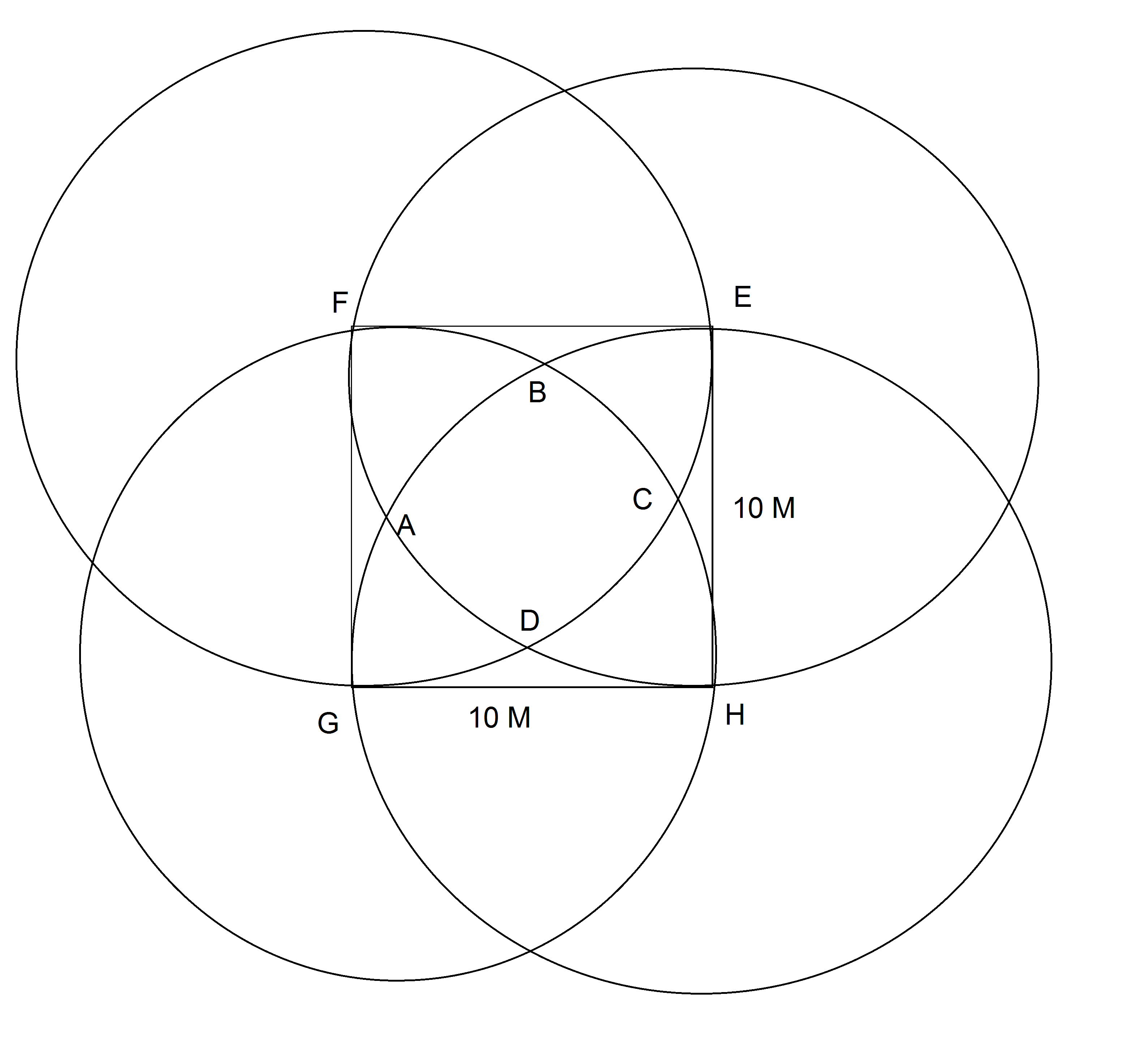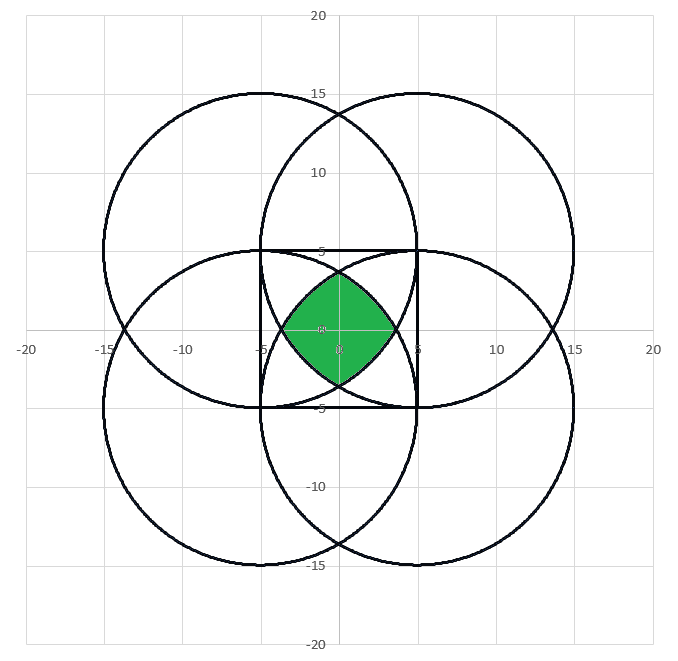# How to resolve this square/circumference problem?

So, in the imagine you have a 10x10 square (EFGH), and E,F,G & H are the centers of their respective circumferences. So, what is the area of ABCD? In meters2.Note by J. P. C. M.
1 month ago

MarkdownAppears as
*italics* or _italics_ italics
**bold** or __bold__ bold
- bulleted- list
• bulleted
• list
1. numbered2. list
1. numbered
2. list
Note: you must add a full line of space before and after lists for them to show up correctly
paragraph 1paragraph 2

paragraph 1

paragraph 2

[example link](https://brilliant.org)example link
> This is a quote
This is a quote
    # I indented these lines
# 4 spaces, and now they show
# up as a code block.

print "hello world"
# I indented these lines
# 4 spaces, and now they show
# up as a code block.

print "hello world"
MathAppears as
Remember to wrap math in $$...$$ or $...$ to ensure proper formatting.
2 \times 3 $$2 \times 3$$
2^{34} $$2^{34}$$
a_{i-1} $$a_{i-1}$$
\frac{2}{3} $$\frac{2}{3}$$
\sqrt{2} $$\sqrt{2}$$
\sum_{i=1}^3 $$\sum_{i=1}^3$$
\sin \theta $$\sin \theta$$
\boxed{123} $$\boxed{123}$$

Sort by:

It is easy by construction sir , by extending the vertices to join points ABCD to form equilateral triangles and by that all the areas we wanted . as such subtracting area of 60° sector from triangle would give symmetrical parts area . now just calculate the length of the square formed inside the bigger square.

- 1 month ago

Are you calculating the green area?- 1 month ago

Try to use Google translate in this site and you will se a easier way to solve it. https://www.tutorbrasil.com.br/questoes-resolvidas/exercicios-resolvidos/arcos-no-quadrado/

- 1 month ago

Exactly same my way I explained above , using equilateral triangles , actually simplifies this problem

- 1 month ago

Yes, I think that Steven Chase made a overcomplicated calculus.

- 1 month ago

The calculus way is much simpler conceptually, but it requires computation. Just depends on how you define "simple".

- 1 month ago

This would make for an excellent problem in the Geometry or Calculus section.

- 1 month ago

The most elementary way (in my opinion) of viewing it is the following:

$A = \int_{-5}^5 \int_{-5}^5 \, M(x,y) \, dx \, dy \\ M(x,y) = 1 \, \text{if} \, (x,y) \, \text{inside all four circles} \\ M(x,y) = 0 \, \text{otherwise}$

- 1 month ago

There is a simple way to explain what you did? A that a 13 year old can understand...

- 1 month ago

Divide the big square into many tiny squares (arranged like a grid). Add up the areas of all of the tiny squares that happen to fall inside all four circles simultaneously. This is difficult to do by hand (I think), but easy to do with a computer.

- 1 month ago